# Test: Single Correct MCQs: Electrostatics | JEE Advanced

## 34 Questions MCQ Test Physics 35 Years JEE Main & Advanced Past year Papers | Test: Single Correct MCQs: Electrostatics | JEE Advanced

Description
Attempt Test: Single Correct MCQs: Electrostatics | JEE Advanced | 34 questions in 70 minutes | Mock test for JEE preparation | Free important questions MCQ to study Physics 35 Years JEE Main & Advanced Past year Papers for JEE Exam | Download free PDF with solutions
QUESTION: 1

### A hollow metal sphere of radius 5 cms is  charged such that the potential on its surface is 10 volts. The  potential at the centre of the sphere is

Solution:

The potential at the surface of a hollow or conducting sphere is same as the potential at the centre of the sphere and any point inside the sphere.

QUESTION: 2

### Two point charges +q and –q are held fixed at (–d, o) and (d, o) respectively of a x-y coordinate system. Then

Solution:

The two charges form an electric dipole. If  we take a point M on the X-axis as shown in the figure, then the net electric field is in –X-direction.

∴ Option (a) is incorrect.
If we take a point N on Yaxis, we find net electric field along  + X direction.
The same will be true for any point on Y-axis. (b) is a correct option.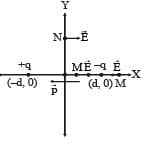NOTE : For any point on the equatorial line of a dipole, the electric field is opposite to the direction of dipole moment.

(b) W∞ – 0 = q (V–V0) = q (0 – 0) = 0
∴ (c) is incorrect. The direction of dipole moment is from –ve to + ve. Therefore (d) is incorrect.

QUESTION: 3

### A parallel plate capacitor of capacitance C is connected to a battery and is charged to a potential difference V. Another capacitor of capacitance 2C is similarly charged to a potential difference 2V. The charging battery is now disconnected and the capacitors are connected in parallel to each other in such a way that the positive terminal of one is connected to the negative terminal of the other. The final energy of the configuration is

Solution:

C and 2C are in parallel to each other.

∴ Resultant capacity = (2C + C)
CR = 3C
Net potential = 2V – V
VR = V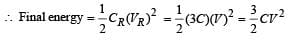QUESTION: 4

Two identical metal plates are given positive charges Q1 and Q2 (<Q1) respectively. If they are now brought close together to form a parallel plate capacitor with capacitance C, the potential difference between them is

Solution:

Within the capacitor,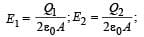where  A = area of each plate
d = separation between two plate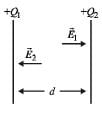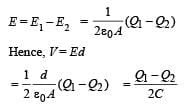QUESTION: 5

For the circuit shown in Figure, which of the following statements is true?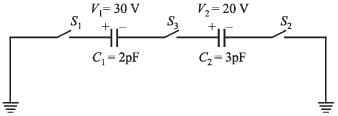Solution:

With the closing of switch  S3 and S1 the negative charge on C2 will attract the positive charge on C2 thereby maintaining the negative charge on C1. The negative charge on C1 will attract the positive charge on C1. No transfer of charge will take place. Therefore p.d across C1 and C2 will be 30 V and 20 V.

QUESTION: 6

Three charges Q, +q and +q are placed at the vertices of a right-angled isosceles triangle as shown. The net electrostatic energy of the configuration is zero if Q is equal to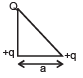Solution: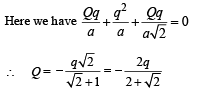QUESTION: 7

A parallel plate capacitor of area A, plate separation d and capacitance C is filled with three different dielectric materials having dielectric constants k1, k2 and k3 as shown. If a single dielectric material is to be used to have the same capacitance C in this capacitor, then its dielectric constant k is given by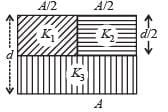Solution: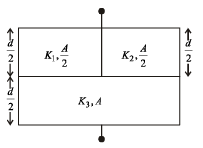Let C1 = Capacity of capacitor with K1
C2 = Capacity of capacitor with K2
C3 = Capacity of capacitor with K3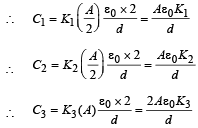C1 and C2 are in parallel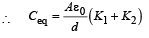Ceq and C3 are in series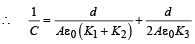But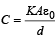for single equivalent capacitor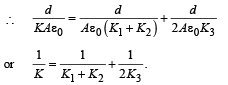QUESTION: 8

Three positive charges of equal value q are placed at the vertices of an equilateral triangle. The resulting lines of force should be sketched as in

Solution:

Electric field lines do not form closed loops. Therefore options (a), (b) and (d) are wrong.
Option (c) is correct. There is repulsion between similar charges.

QUESTION: 9

Consider the situation shown in the figure. The capacitor A has a charge q on it whereas B is uncharged. The charge appearing on the capacitor B a long time after the switch is closed is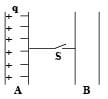Solution:

When S is closed, there will be no shifting of negative charge from plate A to B as the charge – q is held by the charge + q. Neither there will be any shifting of charge from B to A.

QUESTION: 10

A uniform electric field pointing in positive x-direction exists in a region. Let A be the origin, B be the point on the x-axis at x = +1 cm and C be the point on the y-axis at y = +1 cm. Then the potentials at the points A, B and C satisfy:

Solution:

NOTE :  As we move along the direction of electric field the potential decreases.

∴ VA > VB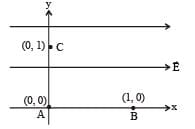QUESTION: 11

Two equal point charges are fixed at x = – a and x = + a on the x-axis. Another point charge Q is placed at the origin.
The change in the electrical potential energy of Q, when it is displaced by a small distance x along the x-axis, is approximately proportional to

Solution:

Initial energy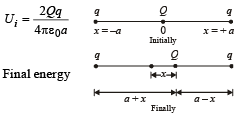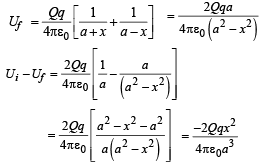when x << a then x2 is neglected in denominator..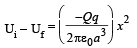QUESTION: 12

Two identical capacitors, have the same capacitance C. One of them is charged to potential V1 and the other V2. The negative ends of the capacitors are connected together.
When the positive ends are also connected, the decrease in energy of the combined system is

Solution:

Initially we know that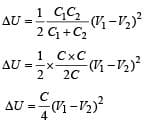QUESTION: 13

A metallic shell has a point charge ‘q’ kept inside its cavity. Which one of the following diagrams correctly represents the electric lines of forces?

Solution:

Electric field everywhere inside the metallic portion of shell is zero.
Hence options (a) and (d) are incorrect.
Electric field lines are always normal to a surface. Hence option (b) is incorrect. Only option (c) represents the correct answer.

QUESTION: 14

Six charges of equal magnitude, 3 positive and 3 negative are to be placed on PQRSTU corners of a regular hexagon, such that field at the centre is double that of what it would have been if only one +ve charge is placed at R. Which of the following arrangement of charge is possible for P, Q, R, S, T and U respectively.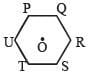Solution: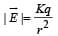Electric field due to P on O is cancelled by electric field due to S on O.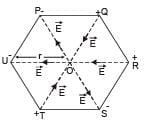to Q on O is cancelled by electric field due to T and O.
The electric field due to R on O is in the same direction as that of U on O.
Therefore the net electric field is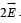QUESTION: 15

A Gaussian surface in the figur e is shown by dotted line. The electric field on the surface will be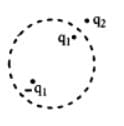Solution:

The flux through the Gaussian surface is due to the charges inside the Gaussian surface. But the electric field on the Gaussian surface will be due to the charges present in side the Gaussian surface and outside it. It will be due to all the charges.

QUESTION: 16

Three infinitely long charge sheets are placed as shown in figure. The electric field at point P is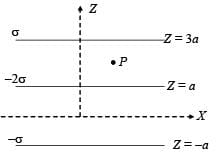Solution:

Figure shows the electric fields due to the sheets 1, 2 and 3 at point P. The direction of electric fields are according to the charge on the sheets (away from positively charge sheet and towards the negatively charged sheet and perpendicular).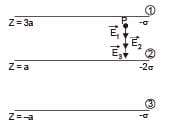The total electric field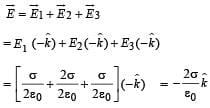QUESTION: 17

A long, hollow conducting cylinder is kept coaxially inside another long, hollow conducting cylinder of larger radius.
Both the cylinders are initially electrically neutral.

Solution:

When a charge density is given to the inner cylinder, the potential developed at its surface is different from that on the outer cylinder. This is because the potential decreases with distance for a charged conducting cylinder when the point of consideration is outside the cylinder.
But when a charge density is given to the outer cylinder, it will change its potential by the same amount as that of the inner cylinder. Therefore no potential difference will be produced between the cylinders in this case.

QUESTION: 18

Consider a neutral conducting sphere. A positive point charge is placed outside the sphere. The net charge on the sphere is then

Solution: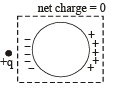When a positive point charge is placed outside a conducting sphere, a rearrangement of charge takes place on the surface.
But the total charge on the sphere is zero as no charge has left or entered the sphere.

QUESTION: 19

A spherical portion has been removed from a solid sphere having a charge distributed uniformly in its volume as shown in the figure. The electric field inside the emptied space is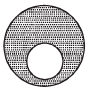Solution: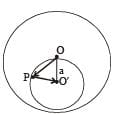Let us consider a uniformly charged solid sphere without any cavity. Let the charge per unit volume be σ and O be the centre of the sphere. Let us consider a uniformly charged sphere of negative charged density s having its centre at O'. Also let OO' be equal to a.

Let us consider an arbitrary point P in the small sphere.
The electric field due to charge on big sphere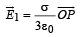Also the electric field due to small sphere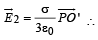The total electric field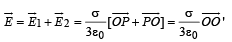Thus electric field will have a finite value which will be uniform.

QUESTION: 20

Positive and negative point charges of equal magnitude are kept at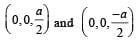respectively. The work done by the electric field when another positive point charge is moved from (–a, 0, 0) to (0, a, 0) is

Solution:

The charges make an electric dipole. A and B points lie on the equatorial plane of the dipole.
Therefore, potential at A = potential at B = 0

W = q (VA – VB) = q × 0 = 0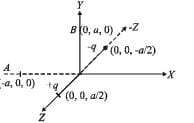QUESTION: 21

Consider a system of three charges q/3, q/3 and –2q/3 placed at points A, B and C, respectively, as shown in the figure.
Take O to be the centre of the circle of radius R and angle CAB = 60°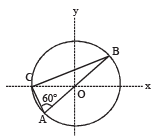Solution:

The electric field due to A and B at O are equal and opposite producing a resultant which is zero. The electric field at O due to C is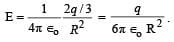∴ Option [A] is not correct. The electric potential at O is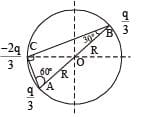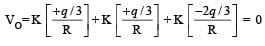∴ Option [D] is wrong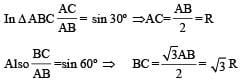Potential energy of the system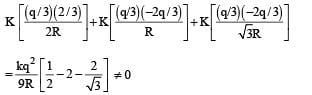∴ Option [B] is wrong Magnitude of force between B and C is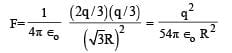QUESTION: 22

A parallel plate capacitor C with plates of unit area and separation d is filled with a liquid of dielectric constant K = 2. The level of liquid is d/3 initially. Suppose the liquid level decreases at a constant speed v, the time constant as a function of time t is –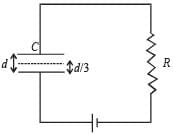Solution:

Let the level of liquid at an instant of time 't' be x. Then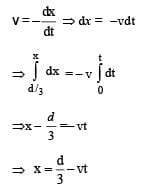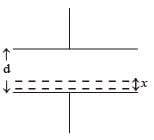Also the capacitance can be considered as an equivalent of two capacitances in series such that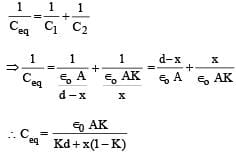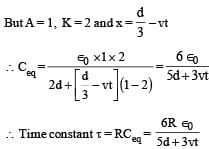QUESTION: 23

Three concentric metallic spherical shells of radii R,  2R, 3R, are given charges Q1, Q2, Q3, respectively. It is found that the surface charge densities on the outer surfaces of the shells are equal. Then, the ratio of the charges given to the shells, Q1 : Q2 : Q3, is

Solution:

The charges on the surfaces of the metallic spheres are shown in the diagram. It is given that the surface charge densities on the outer surfaces of the shells are equal.
Therefore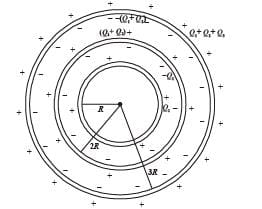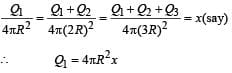Q1 + Q2 = 4π(2R)2k = 4[4πR2x]

⇒ Q2 = 4[4πR2 x] - Q1

= 4[4πR2x] -4πR2x = 3[4πR2x]
Also Q1 + Q2+ Q3 = 4π(3R)2 x = 9[4πR2x]
∴ Q3 = 9[4πR2x] - Q1 - Q2 = 9[4πR2x] - [4πR2x] -3[4πR2x] = 5[4πR2x]

⇒ Q1 : Q2 :Q3 = 1 :3 :5

QUESTION: 24

A disc of radius a / 4 having a uniformly distributed charge 6C is placed in the x - y plane with its centre at (–a / 2, 0, 0).
A rod of length a carrying a uniformly distributed charge 8C is placed on the x - axis from x = a /4 to x = 5a / 4. Two point charges – 7 C and 3 C are placed at (a / 4, – a /4, 0) and (– 3a /4, 3a / 4, 0), respectively. Consider a cubical surface formed by six surfaces x = ± a / 2, y = ± a / 2, z = ± a / 2. The electric flux through this cubical surface is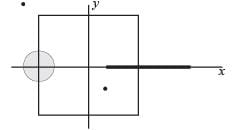Solution:

From the figure it is clear that the charge enclosed in the cubical surface is 3C + 2C – 7C = –2C. Therefore the electric flux through the cube is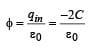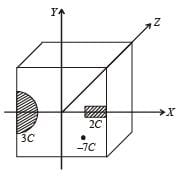QUESTION: 25

A uniformly charged thin spherical shell of radius R carries uniform surface charge density of σ per unit area. It is made of two hemispherical shells, held together by pressing them with force F (see figure). F is proportional to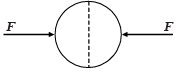Solution:

The electrostatic pressure at a point on the surface of a uniformly charged sphere  =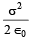∴ The force on a hemispherical shell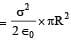QUESTION: 26

A tiny spherical oil drop carrying a net charge q is balanced in still air with a vertical uniform electric field of strength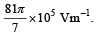When the field is switched off, the drop is observed to fall with terminal velocity 2 ×10–3 ms –1. Given g = 9.8 m s–2, viscosity of the air = 1.8 × 10–5 Ns m–2 and the density of oil = 900 kg m–3, the magnitude of q is

Solution:

When the electric field is on
Force due to electric field = weight

qE = mg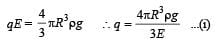When the electric field is switched off Weight = viscous drag force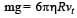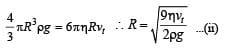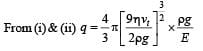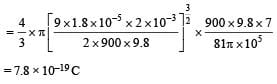QUESTION: 27

Consider an electric field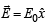where E0 is a constant.
The flux through the shaded area (as shown in the figure) due to this field is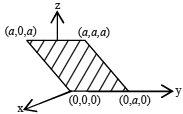Solution: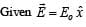This shows that the electric field acts along + x direction and is a constant. The area vector makes an angle of 45° with the electric field. Therefore the electric flux through the shaded portion whose area is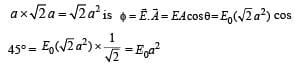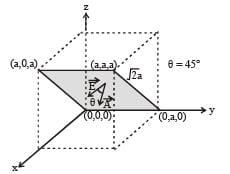QUESTION: 28

A 2 μF capacitor is charged as shown in the figure. The percentage of its stored energy dissipated after the switch S is turned to position 2 is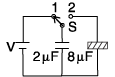Solution:

When S and 1 are connected

The 2μF capacitor gets charged. The potential difference across its plates will be V.
The potential energy stored in 2 μF capacitor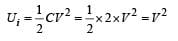When S and 2 are connected The 8mF capacitor also gets charged. During this charging process current flows in the wire and some amount of energy is dissipated as heat. The energy loss is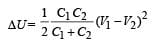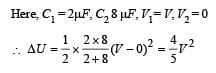The percentage of the energy dissipated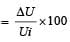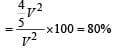QUESTION: 29

Which of the field patterns given below is valid for electric field as well as for magnetic field?

Solution:

The pattern of field lines shown in option (c) is correct because
(a) a current carrying toroid produces magnetic field lines of such pattern
(b) a changing magnetic field with respect to time in a region perpendicular to the paper produces induced electric field lines of such pattern.

QUESTION: 30

A wooden block performs SHM on a frictionless surface with frequency, v0. The block carries a charge +Q on its surface. If now a uniform electric field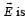switched-on as shown, then the SHM of the block will be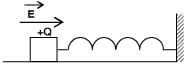Solution:

The frequency of SHM performed by wooden block is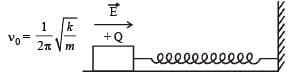when electric field is switched on, the value of k and m is not affected and therefore the frequency of SHM remains the same. But as an external force QE starts acting on the block towards right, the mean position of SHM shifts towards right by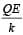correct option is (a).
Note : In SHM if a constant additional force is applied then it only shift the quilibrium position and does not change the frequency fo SHM.

QUESTION: 31

Two large vertical an d parallel metal plates having a separation of 1 cm are connected to a DC voltage source of potential difference X. A proton is released at rest midway between the two plates. It is found to move at 45° to the vertical JUST after release. Then X is nearly

Solution:

The two forces acting on the proton just after the release are shown in the figure. In this situation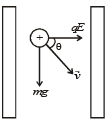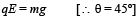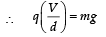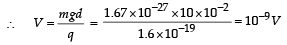QUESTION: 32

Consider a thin spherical shell of radius R with centre at the origin, carrying uniform positive surface charge density. The variation of the magnitude of the electric field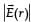and the electric potential V(r) with the distance r from the centre, is best represented by which graph?

Solution:

For a thin uniformly positive charged spherical shell

(i) Inside the shell at any point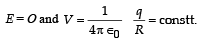where q = charge on sphere

(ii) Outside the shell at any point at any distance r from the centre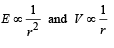QUESTION: 33

In the given circuit, a charge of +80 μC is given to the upper plate of the 4 μF capacitor. Then in the steady state, the charge on the upper plate of the 3 μF capacitor is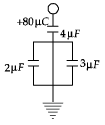Solution:

The total charge on plate A will be – 80 µC. If qB and qC be the charges on plate B and C then

qB + qC = 80 µC ...(1)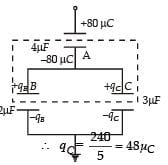Also 2µF and 3µF capacitors are in parallel. Therefore,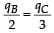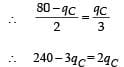This charge will obviously be positive.

QUESTION: 34

Charges Q, 2Q and 4Q are uniformly distributed in three dielectric solid spheres 1, 2 and 3 of radii R/2, R and 2R respectively, as shown in figure. If magnitude of the electric fields at point P at a distance R from the centre of sphere 1, 2 and 3 are E1, E2 and E3 respectively, then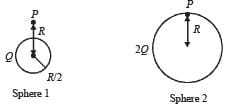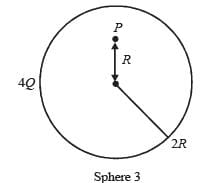Solution: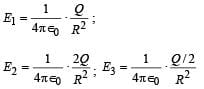Clearly E2 > E1 > E3 where Q/2 is the charge enclosed in a sphere of radius R concentric with the given sphere.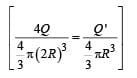Use Code STAYHOME200 and get INR 200 additional OFF Use Coupon Code